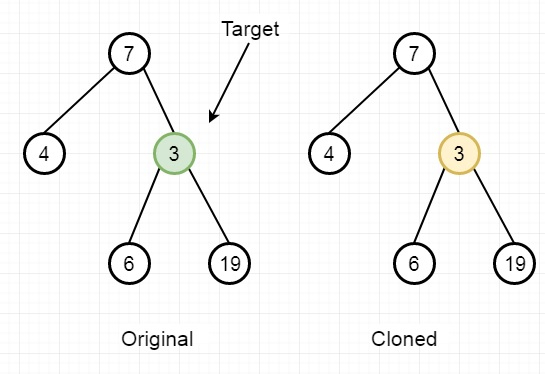# Find a Corresponding Node of a Binary Tree in a Clone of That Tree in C++

Suppose we have two binary trees original and cloned and given a reference to a node target in the original tree. The cloned tree is actually a copy of the original tree. We have to find a reference to the same node in the cloned tree.

So if the tree is like below and the target is 3, then the output will be 3.To solve this, we will follow these steps −

• Define a method called solve(), this will take the node1m node2 and target

• if node1 is null, then return null

• if node1 is target and value of node 1 is the value of node2, then return node2

• leftPart := solve(left of node1, left of node2, target)

• rightPart := solve(right of node1, right of node2, target)

• return leftPart, if leftPart is not null, otherwise rightPart

• From the main method call return solve(original, cloned, target)

## Example (C++)

Let us see the following implementation to get a better understanding −

Live Demo

#include <bits/stdc++.h>
using namespace std;
class TreeNode{
public:
int val;
TreeNode *left, *right;
TreeNode(int data){
val = data;
left = right = NULL;
}
};
void insert(TreeNode **root, int val){
queue<TreeNode*> q;
q.push(*root);
while(q.size()){
TreeNode *temp = q.front();
q.pop();
if(!temp->left){
if(val != NULL)
temp->left = new TreeNode(val);
else
temp->left = new TreeNode(0);
return;
} else {
q.push(temp->left);
}
if(!temp->right){
if(val != NULL)
temp->right = new TreeNode(val);
else
temp->right = new TreeNode(0);
return;
} else {
q.push(temp->right);
}
}
}
TreeNode *make_tree(vector<int> v){
TreeNode *root = new TreeNode(v);
for(int i = 1; i<v.size(); i++){
insert(&root, v[i]);
}
return root;
}
class Solution {
public:
TreeNode* solve(TreeNode* node1, TreeNode* node2, TreeNode*
target){
if(!node1) return NULL;
if(node1 == target && node1->val == node2->val) return node2;
TreeNode* leftPart = solve(node1->left, node2->left, target);
TreeNode* rightPart = solve(node1->right, node2->right, target);
return leftPart? leftPart : rightPart;
}
TreeNode* getTargetCopy(TreeNode* original, TreeNode* cloned,
TreeNode* target) {
return solve(original, cloned, target);
}
};
main(){
vector<int> v = {7,4,3,NULL,NULL,6,19};
TreeNode *root = make_tree(v);
TreeNode *cloned = make_tree(v);
TreeNode *target = root->right; //The node with value 3
Solution ob;
cout << (ob.getTargetCopy(root, cloned, target))->val;
}

## Input

[7,4,3,null,null,6,19]
3

## Output

3